2006/02 Research & Review

# Test of the Efficiency Structure Hypothesis with an Application to Major Japanese Banks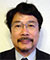TSUTSUI Yoshiro
Faculty Fellow, RIETI and Professor, Institute of Social & Economic Research, Osaka University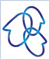SATAKE Mitsuhiko
Professor, Ryukoku UniversityUCHIDA Hirofumi
Associate Professor, Wakayama University

## Introduction

The purpose of this paper is to examine whether the efficiency structure hypothesis holds true for major Japanese commercial banks. The efficiency structure hypothesis, developed by Demesetz (1973), is the proposition that more efficient companies will better compete, develop and grow in scale, thus resulting in an increase in the degree of market concentration. It also assumes that such companies will achieve high profitability while maintaining high market shares. Hence, under this hypothesis, it is expected that the greater the degree of market concentration, the more efficient the market.

This paper attempts to examine the efficiency structure hypothesis by using a direct method that focuses on a basic proposition independent from the structure-conduct -performance (SCP) hypothesis. The basic proposition is that "more efficient companies win competition and grow." In this paper, we test this relationship between the efficiency and growth of companies by first measuring inefficiency through estimation of the cost function and then examining the impact of such indicators on the degree of growth of companies.

## Models

The analysis presented in this paper takes a two-step approach. The first step is to estimate the efficiency of banks, and the second to examine how it affects the subsequent growth of the banks.

(1) Estimation of efficiency
There are several methods for estimating efficiency. In this paper we use the stochastic frontier function approach. We use loans L as an output of banks. As input prices, only the wage rate w is taken into account whereas prices of capital equipment are excluded, assuming that all the major commercial banks face an identical price of capital equipment. Thus, we estimate the following translog cost function: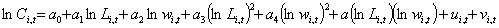(i)

where InL and lnw stands for deviations from their means.

In this paper, we assume that the indicator of inefficiency u is determined by the mechanism described in equation (ii) below, and estimate eqs. (i) and (ii) simultaneously.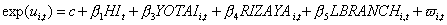(ii)

where HI represents the Herfindahl index, YOTAI the ratio of loans to deposits, RIZAYA the interest rate spread, and LBRANCH the logarithm of the number of branches.

The estimates of inefficiency u, is to measure the so-called X-inefficiency. However, u alone is not enough to capture the total efficiency of a bank. Thus, in addition to u, the scale elasticity SE, as defined by the equation (iii), will be used to examine how the efficiency of banks impacts their future growth. SE, as referred to here, represents the scale diseconomy.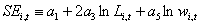(iii)

(2) Testing of the efficiency structure hypothesis
In this paper, it is assumed that the amount of loans is determined in the way that demand matches supply. For the supply of loans, we assume the loan supply function applicable to an oligopolistic bank under the usual static profit maximization model. Therefore, the supply of loans depends on the loan interest rate, the interest rate of the substitutable assets, specifically the call rate rc, and variables representing competition in the market (conjectural variations of other banks and demand elasticity; HI is used here), and also -- when the operating cost function depends on the product of the amount of loans and size of bank -- on variables indicating the size of banks (the amount of deposits is used here). Furthermore, we assume that the supply of loans is positively influenced by the capital ratio CR because banks consider their default risks. On the basis of these assumptions, we test the hypothesis that the greater a bank's efficiency in the previous year, the greater the amount of loans supplied by the bank in the current year. Here, we examine the impact of two kinds of efficiency, organizational inefficiency and the diseconomies of scale.

Meanwhile, we assume that the demand for loans depends on the loan interest rates and variables indicating the scale of demanders (GDP is used here). The following reduced-form function for loan is derived by solving the demand and supply functions for loans: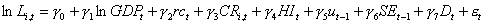(iv)

Hypotheses we are focusing on are as follows:

<Hypothesis 1>
The greater a bank's organizational efficiency, the greater the amount of loans in the next year. In equation (iv), γ5 < 0.

<Hypothesis 2>
The greater a bank's scale efficiency, the greater the amount of loans in the next year. In equation (iv), γ6 < 0.

Other coefficients are expected to be γ1 > 0, γ2 <0, γ3 >0, γ7 >0. If it is assumed that the more monopolistic the market, the lower the amount of loans provided, γ4 < 0.

## Estimation results

This paper analyzes the performance of major commercial banks and long-term credit banks during fiscal years 1974-2001.

When equations (i) and (ii) are simultaneously estimated, all the coefficients in the equation (i) are significant, among which only the cross term coefficient a5 is negative and all the others positive. The scale elasticity evaluated in average is a1, which takes on 0.536 meaning large economies of scale.

The results of the estimation of equation (iv) by using the above estimate as the indicator of organizational inefficiency are shown in Table 1. As shown, organizational efficiency, which is the focused variable, is negative but not significant. On the other hand, the diseconomy of scale is significantly positive. These results are opposite to the efficiency structure hypothesis which predicts that the smaller the economies of scale, the greater the amount of loans advanced in the next year.

Table 1: Estimation results of equation (iv)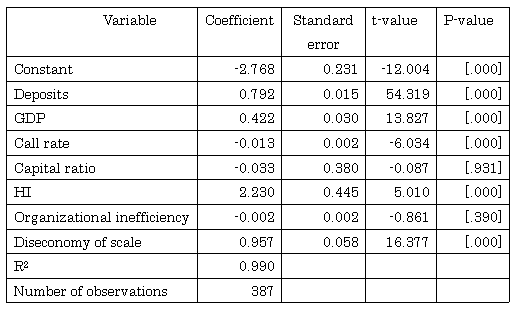## Analysis using assets as the scale of a bank

In the preceding section, we adopt the amount of loans as the scale of a bank. However, in that model, we assume that banks determine the amount of loans so as to maximize their profits considering of the budget constraints, where the amount of deposits is treated as an exogenous variable, meaning that the model is testing whether banks increase the amount of loans relative to the amount of deposits as given. This may be different from the efficiency structure hypothesis we want to examine. Our purpose is to examine whether or not efficient banks grow in absolute size. In this section, we analyze by using the amount of assets -- instead of loans -- as the primary indicator of bank size, and then, examine the results.

We assume the following equation, replacing the equation (i):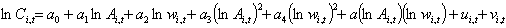(i)'

We assume the following equation, replacing the equation (ii):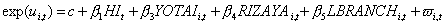(ii)'

Here, lnA represent deviations from the mean. Economies of scale can be calculated by the following equation: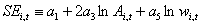(iii)'

We estimate the following equation, instead of equation (iv):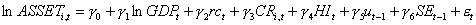(iv)'

Using the estimate of the inefficiency, we estimate equation (iv)', the results of which are shown in Table 2. As expected, the coefficient of GDP is positive. Meanwhile, the coefficients for the call rate and capital ratio are negative and positive respectively, both of which are consistent with the expected results but neither is significant. The Herfindahl index (HI), though not significant, is negative, which is consistent with what has been expected in the SCP hypothesis.

What should be noted here is that both the coefficients of organizational inefficiency and the scale elasticity are negative and highly significant. That is, the efficiency structure hypothesis is supported by both of these two indicators.

Table 2: Estimation results of equation (iv)' -- Analysis using assets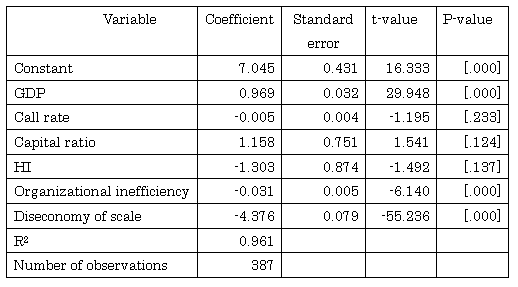## Changes with time

Would the tendency derived from the estimation results in the preceding section differ from one time to another? To find this out, we divide the period of 1974-2001 into three sub-periods -- 1970s, '80s and '90s -- and estimate the equations for each sub-period. The estimation results show that organizational inefficiency is significantly negative and diseconomy of scale significantly positive in all three sub-periods. The value of these two coefficients increases as the time passes. The outcome that the coefficient of organizational inefficiency is negative is consistent with the efficiency structure hypothesis, and the smallest value of the coefficient in the 1970s may be attributable to less competitive pressure at the time. With respect to other variables, it is notable that in the 1990s, the GDP is not significant and its coefficient is smaller. Here we can observe the impact of a decrease in the amount of loans (or the so-called credit crunch) in the 1990s.

The estimation results with assets show that both the organizational inefficiency and diseconomies of scale are significantly negative throughout the three sub-periods, demonstrating that the efficiency structure hypothesis holds true. However, the coefficient of organizational inefficiency is lower in the 1970s and '80s -- as is the case for analysis with the amount of loans -- and the significance is somewhat smaller in the 1970s.

## Conclusion

This paper examines whether the efficiency structure hypothesis holds true for the major Japanese commercial banks, using data covering fiscal years 1974-2001. The efficiency structure hypothesis has been examined mostly in comparison with the SCP hypothesis in the framework that determines which of the two measurements -- the degree of market concentration or market shares -- better explains market performance such as profits and interest rates. In this paper, we point out problems with this framework and examine the efficiency structure hypothesis in a more straightforward way by focusing on the proposition that the more efficient a bank is, the more it grows.

First we estimate organizational inefficiency and the diseconomy of scale for major banks using panel data. Then we examine how these estimates affect the size of banks in the following fiscal year.

In the regression analysis using the reduced-form for loan incorporating organizational inefficiency and the diseconomy of scale in the previous year, we find that the organizational inefficiency has a negative impact but diseconomy of scale, contrary to expectation, has a positive impact. In contrast, the analysis with banks' assets, both the organizational inefficiency and the diseconomy of scale have a negative impact, that is consistent with the efficiency structure hypothesis.

We conduct the same analyses by splitting the observation period into three sub-periods, 1970s, 1980s and 1990s, in which we find that the organizational inefficiency and diseconomy of scale generally remains unchanged. However, closer scrutiny of the outcome reveals that the impact of organizational inefficiency in the 1970s is smaller than its impact in the other two sub-periods. This indicates that the degree of competition among the major Japanese commercial banks was still small in the 1970s, as pointed out in Uchida and Tsutsui (2005).

>> Original text in Japanese

March 6, 2005

## Article(s) by this author

• ### Test of the Efficiency Structure Hypothesis with an Application to Major Japanese Banks

March 6, 2005［Keizai Sangyo Journal］